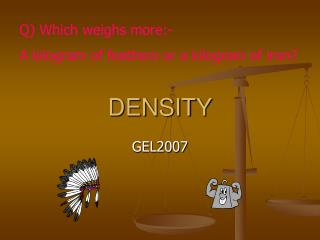DownloadDownload PresentationDENSITY

# DENSITY

Télécharger la présentation## DENSITY

- - - - - - - - - - - - - - - - - - - - - - - - - - - E N D - - - - - - - - - - - - - - - - - - - - - - - - - - -
##### Presentation Transcript

1. Q) Which weighs more:- A kilogram of feathers or a kilogram of iron? DENSITY GEL2007

2. What is Density? If you take the same volume of different substances, then they will weigh different amounts. Density is the Mass per unit Volume Wood Water Iron 1 cm3 1 cm3 1 cm3 IRON 0.50 g 1.00 g 8.00 g Q) Which has the greatest mass and therefore the most dense?

3. m  V Density Equation: g or kg Mass Density = Volume gcm-3 or kgm-3 cm3 or kg3 • = m V Example: Q) Liquid water has a density of 1000kgm-3, while ice has density of 920kgm-3. Calculate the volume occupied by 0.25kg of each. V= m = 0.25 = 0.000250m3  1000 V = m = 0.25 = 0.000272m3  920

4. DENSITY OF A REGULAR SOLID • Find the Mass of the solid on a balance. • Measure the three lengths and calculate the Volume. (ie V = l x w x h ) • Calculate the Density. m = 240 g 2.0 cm = m = 240 =10.0 g/cm3 V 24 3.0 cm 4.0 cm

5. DENSITY OF AN IRREGULAR SOLID m = 360 g  = m = 360 =12.0 g/cm3 V 30 • Find the Mass of the solid on a balance. • Fill the Measuring Cylinder with Water to a known Volume. • Add the Object. • Work out the Volume of Water that is displaced. • Calculate the Density. 80 cm3 50 cm3

6. DENSITY OF AN IRREGULAR SOLID • OR use a Eureka Can to find the Volume. • Find the mass of the solid on a balance. • Add water until just overflowing. • Place a Measuring Cylinder under the spout. • Add the Object. • Collect the Water and read off the Volume. • Calculate Density m = 440 g 40.0 cm3  = m = 440 =11.0 g/cm3 V 40

7. Mass of Liquid = Mass of Measuring Cylinder and Liquid – Mass of empty Measuring Cylinder DENSITY OF A LIQUID • Find the Mass of an empty Measuring Cylinder. • Add a certain Volume of Liquid. • Find the Mass of the Measuring Cylinder and Liquid • Calculate the Mass of Liquid. • How? • Calculate Density of Liquid. 25.0 g 45.0 g 20.0 cm3 45 – 25 = 20 g  = m = 20 =1.00 g/cm3 V 20

8. DENSITY OF A GAS • Remove the air from a flask of a known Volume, using a vacuum pump. • Find its Mass. • Add the gas to be tested. • Reweigh. • The difference is the Mass of gas. • Calculate Density. To vacuum flask 150.0 g 170.0 g 1000 cm3 170 -150 = 20.0g  = m = 20 =0.0200 g/cm3 V 1000